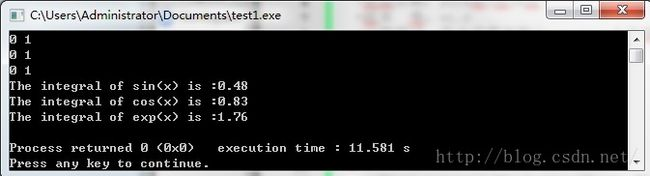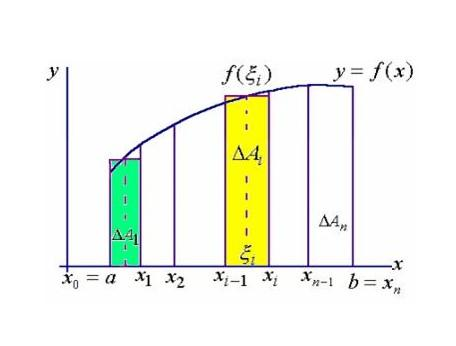• C语言：利用函数指针编写一个用矩形法求定积分的通用函数,包括正弦,余弦和指数函数,
2021-05-21 09:05:58

问题描述：

C语言：利用函数指针编写一个用矩形法求定积分的通用函数,包括正弦,余弦和指数函数,

//我运行的结果和答案对不上,请帮我看看程序对吗?

//实现积分

#include

#include

int main()

{

float fsin(float);

float fcos(float);

float fexp(float);

float fun(float,float,float(*)(float),int);

float a1,b1,a2,b2,a3,b3;

int n=20;

float (*p)(float);

printf("input a1,b1 for sin(x):\n");

scanf("%f%f",&a1,&b1);

printf("input a2,b2 for cos(x):\n");

scanf("%f%f",&a2,&b2);

printf("input a3,b3 for exp(x):\n");

scanf("%f%f",&a3,&b3);

p=fsin;

printf("sin(x)的积分：%f\n",fun(a1,b1,p,n));

p=fcos;

printf("cos(x)的积分：%f\n",fun(a2,b2,p,n));

p=fexp;

printf("exp(x)的积分：%f\n",fun(a3,b3,p,n));

getch();

return 0;

}

float fsin(float x)

{

return sin(x);

}

float fcos(float x)

{

return cos(x);

}

float fexp(float x)

{

return exp(x);

}

float fun(float a,float b,float(*p)(float x),int n)

{

int i;

float x,h,s;

h=(b-a)/n;

x=a;

s=0;

for(i=0;i

1个回答

分类：

综合

2014-12-07

问题解答：

我来补答

for(i=0;i

展开全文阅读

更多相关内容
• 今天小编就为大家分享一篇C语言:利用指针编写程序,用梯形法计算给定的定积分实例，具有很好的参考价值，希望对大家有所帮助。一起跟随小编过来看看吧

/*

*文件名称：test1.cpp

*作 者：刘金石

*完成日期：2016年3月16日

*版本 号：v1.0

*问题描述：写一个用矩形法求定积分的通用函数.

*输入描述：输入定积分的上限和下限。

*输出描述：输出sin(x),cos(x),exp(x)的结果。

*/

#include

#include

using namespace std;

int main()

{

float integral(float (*p)(float),float a,float b,int n);

float a1,b1,a2,b2,a3,b3,c,(*p)(float);

float fsin(float);

float fcos(float);

float fexp(float);

int n=20;

scanf("%f%f",&a1,&b1);

scanf("%f%f",&a2,&b2);

scanf("%f%f",&a3,&b3);

p=fsin;

c=integral(p,a1,b1,n);

printf("The integral of sin(x) is :%.2f\n",c);

p=fcos;

c=integral(p,a2,b2,n);

printf("The integral of cos(x) is :%.2f\n",c);

p=fexp;

c=integral(p,a3,b3,n);

printf("The integral of exp(x) is :%.2f\n",c);

return 0;

}

float integral(float(*p)(float),float a,float b,int n)

{

int i;

float x,h,s;

h=(b-a)/n;

x=a;

s=0;

for(i=1;i<=n;i++)

{

x=x+h;

s=s+(*p)(x)*h;

}

return s;

}

float fsin(float x)

{

return sin(x);

}

float fcos(float x)

{

return cos(x);

}

float fexp(float x)

{

return exp(x);

}

运行结果：展开全文• 二、C语言实现定积分（函数指针的方法）1. 上才（代）艺（码）2.读入数据总结 前言 定积分是什么？定积分是如何计算的？用C语言该如何实现 一、定积分是什么？ 定积分定义： 设函数f(x) 在区间[a,b]上连续，将区间...

# 前言

定积分是什么？定积分是如何计算的？用C语言该如何实现

# 一、定积分是什么？

定积分定义：

设函数f(x) 在区间[a,b]上连续，将区间[a,b]分成n个子区间[x0,x1], (x1,x2], (x2,x3], …, (xn-1,xn]，其中x0=a，xn=b。

可知各区间的长度依次是：△x1=x1-x0，在每个子区间(xi-1,xi]中任取一点ξi（1,2,…,n），作和式 。该和式叫做积分和，设λ=max{△x1, △x2, …, △xn}（即λ是最大的区间长度）。

如果当λ→0时，积分和的极限存在，则这个极限叫做函数f(x) 在区间[a,b]的定积分，记为 ，并称函数f(x)在区间[a,b]上可积。

其中：a叫做积分下限，b叫做积分上限，区间[a, b]叫做积分区间，函数f(x)叫做被积函数，x叫做积分变量，f(x)dx 叫做被积表达式，∫ 叫做积分号。以上解析来自于百度知道

# 二、C语言实现定积分（函数指针的方法）

## 1. 上才（代）艺（码）

代码中有部分注释
代码如下：

/**
* @file MooC_Point_Dingjifen.c
* @author Zheng Hao (2278757316@qq.com)
* @brief 函数指针实现定积分的计算，中国大学Mocc苏小红老师的C语言教程(关于指针)
* @version 0.1
* @date 2022-01-08
*
*
*/
#include <stdio.h>
#include <stdlib.h>
float Integral(float (*f)(float), float a, float b);
float F1(float x);
float F2(float x);
int main()
{
float y1,y2;
y1=Integral(F1,0.0,1.0);
y2=Integral(F2,0.0,3.0);
printf("F1的值：%lf\nF2的值：%lf\n",y1,y2);
//程序中断函数，如果用的编辑器不是VsCode可以忽略
system("pause");
return 0;
}
// 指针调用函数计算定积分
float Integral(float (*f)(float), float a, float b)
{
//S是面积，h指高
float s, h;
int n = 100, i;
s = ((*f)(a) + (*f)(b)) / 2;
h = (b - a) / n;
for (i = 1; i <=n; i++)
{
s = s + (*f)(a + i * h);
}
return s * h;
}
float F1(float x)
{
//计算函数1/(1+X^2)
return 1+x*x;
}
float F2(float x)
{
//计算函数
return x/(1+x*x);
}


## 2.实现原理

这里主要是给大家看调试图。# 总结

注意观察上图中左上方的监视值。这里计算定积分的方法是用到了定积分的几何意义来计算。用到的知识有：指针函数的用法、用到的编辑器是VSCode。
下面是网课的链接地址，大家感兴趣可以看一下。
C语言设计精髓

展开全文• ## C语言求定积分

千次阅读 2019-03-13 16:49:42
那现在就用这种方法，利用C语言定积分。 下面附上代码： #include &amp;lt;stdio.h&amp;gt; #include &amp;lt;stdlib.h&amp;gt; #include &amp;lt;iostream&amp;gt; #include &...

这里以函数f=sinx为例子，首先思路：求定积分用的是元素法，把面积分为一个个的小矩形，分的越密集，则结果越正确。那现在就用这种方法，利用C语言求定积分。
下面附上代码：

#include <stdio.h>
#include <stdlib.h>
#include <iostream>
#include <string.h>
#include <math.h>
using namespace std;
int main()
{
int i;
double a,b;
double wide=0.001;//wide表示划分的单位宽度，wide越小结果越精确，n是积分中矩形的宽
scanf("%lf%lf",&a,&b);
int n=(b-a)/wide;//a，b为积分的上限和下限
double s=0;//s为所有小矩形面积的和，即积分的值
for(i=0; i<n; i++)
{
s+=sin(a+i*wide)*wide;//a+i*wide是横坐标的值
}
printf("%.2lf\n",s);
return 0;
}


下面做一下升级，方便函数变化时，灵活运用。

#include <stdio.h>
#include <stdlib.h>
#include <iostream>
#include <string.h>
#include <math.h>
using namespace std;
double fun(double x)
{
return sin(x);
}
double jifen(double a,double b,double (*p)(double))//(*p)(double)为指向函数的指针，相当于一个函数的名字作为形参了
{
int i;
double wide=0.001;//wide表示划分的单位宽度，wide越小结果越精确，n是积分中矩形的宽
dint n=(b-a)/wide;//a，b为积分的上限和下限
double s=0;//s为所有小矩形面积的和，即积分的值
for(i=0;i<n;i++)
{
s+=(*p)(a+i*wide)*wide;//a+i*wide是横坐标的值
}
return s;
}
int main()
{

double a,b;
scanf("%lf%lf",&a,&b);
printf("%.2lf\n",jifen(a,b,fun));
return 0;
}



注意：double (*p)(double)表示p是指向函数的指针，被指的函数是一个实型函数，有一个实型的形参，p是指向函数的指针变量。同以往的指针不同的是，p指向的是一个函数，代表一个函数的地址，p就代表这个函数的名字。(p)(a+widei)相当于sin(a+widei)。这样jifen函数就是一个通用求定积分的函数了。里面的函数定义随意变，而所需改变的代码很少。这样大大提高了效率

展开全文• 用矩形法分别求函数y = sin(x) 在函数[0,1]区间的定积分、y = cos(x)在 [-1, 1]区间的定积分、y = 5x^2^ + 6x + 7在 [1, 3] 区间的定积分，要求编程时使用指向函数的指针变量。 #define _CRT_SECURE_NO_WARNINGS #...
• 本文为大家分享了C语言使用矩形法求定积分的通用函数，供大家参考，具体内容如下要求：写一个用矩形法求定积分的通用函数，分别求：sin(x),cos(x),e^x 。分析：矩形法，学过高等数学就知道化曲为直的思想。将定积分...
• 根据定积分的定义及几何意义，定积分就是求函数fx在区间,ab中图线下包围的面积。将积分区间n等分，各子区间的面积近似等于梯形的面积，面积的计算运用梯形公式求解，再累加各区间的面积，所得的和近似等于被...
• 利用指针编写程序，用梯形法计算下列公式中的定积分： ∫100(x3+12x+1)dx∫010(x3+12x+1)dx\int_0^{10} {\left( {{x^3} + \frac{1}{2}x + 1} \right)} dx 参考代码 首先说明一下指针的用处：因为所传递的参数均为...
• 本文分别介绍定积分的近似计算的三种方法：矩形法、梯形法、抛物线法。开发语言 算法
• 今天是进筑梦实验室的第一天，心里很...例题：用指针的方法将两个数按照从大到小的顺序输出 在做这个题的时候，一开始把主函数写在了前面，然后一直是编译错误；但是我把主函数放前面就是对的，认为是我函数声明有误开发语言 后端
• 利用函数指针变量编写一个求定积分的通用函数，答案:4信息版本：手机版解决时间 2021-05-05 09:17已解决2021-05-05 02:15用它分别求5个函数的定积分:每次需要求定积分的函数是不一样的。可以编写一个求定积分的通用...
• #include"integral.h"double integral::definiteIntegral(double(*f)(double), double a, double b, double step)//函数功能：计算函数在[a,b]的定积分//参数1：函数指针；参数2：积分上限；参数3：积分下限；参数4...
•算法
• 2015-01-22 06:30:01阅读( 513 )解： #includemain(){ float integral(float(8p)(float),float a,float b,int n);float a1,b1,a2,b2,a3,b3,c,(*p)(float);float fsin(float);float fcos(float);...
• 指向函数的指针用函数指针变量调用函数例子1定义和使用指向函数的指针变量例子2用指向函数的指针做函数参数例子3练习 不止数，字符串以及数组有首地址，函数也有首地址。编译时，内存给每一个函数留出空间来储存函数...
• 例题：编写一个用矩形求定积分的通用库函数。 p.s.这个只是近似值。。。 代码如下 #include&amp;lt;math.h&amp;gt; #include&amp;lt;stdio.h&amp;gt; double integral(double(*fun)(),double a,...
• 写一个用矩形法求定积分的通用函数，分别求：sin(x),cos(x),e^x 。 分析： 矩形法，学过高等数学就知道化曲为直的思想。将定积分化为多个函数连续的和。基本思想是将区间[a，b]化成n等分，当n越大的时候结果越准确...数学
• 1.用 ”定积分定义“ 得出积分数值以及 ”牛顿-莱布尼兹公式“ (微积分基本定理)得出数值的差异。 2.用定积分定义积分，”分段点“数量越多趋向于无穷个时—— 即n越大，趋向于无穷的时候，用 "定积分定义" 积...
• 按如下函数原型，采用梯形法编程实现，在积分区间[a,b]内计算函数y1=1+x²在[0,1]的定积分和函数y2=在[0,3]的定积分。其中，指向函数的指针变量f用于接收被积函数的入口地址 #include<stdio.h> float ...算法...